# WO2019009622A1 - Intra-prediction mode-based image processing method and apparatus therefor - Google Patents

## Info

Publication number
WO2019009622A1
WO2019009622A1 PCT/KR2018/007590 KR2018007590W WO2019009622A1 WO 2019009622 A1 WO2019009622 A1 WO 2019009622A1 KR 2018007590 W KR2018007590 W KR 2018007590W WO 2019009622 A1 WO2019009622 A1 WO 2019009622A1
Authority
WO
WIPO (PCT)
Prior art keywords
sample
reference sample
current block
prediction
lower
Prior art date
Application number
PCT/KR2018/007590
Other languages
French (fr)
Korean (ko)
Inventor
허진
유선미
Original Assignee
엘지전자 주식회사
Priority date (The priority date is an assumption and is not a legal conclusion. Google has not performed a legal analysis and makes no representation as to the accuracy of the date listed.)
Filing date
Publication date
Priority to US201762528445P priority Critical
Priority to US62/528,445 priority
Application filed by 엘지전자 주식회사 filed Critical 엘지전자 주식회사
Publication of WO2019009622A1 publication Critical patent/WO2019009622A1/en

• 238000000034 methods Methods 0 description 30
• 239000002609 media Substances 0 description 13
• 230000001131 transforming Effects 0 description 4
• 238000006243 chemical reaction Methods 0 description 3
• 238000004891 communication Methods 0 description 3
• 230000000694 effects Effects 0 description 2
• 239000000203 mixtures Substances 0 description 1

## Images

•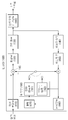•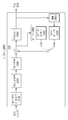•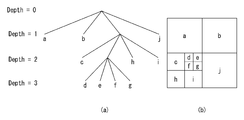•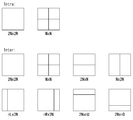•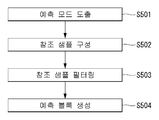•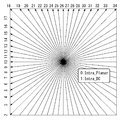•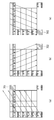•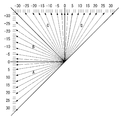•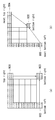•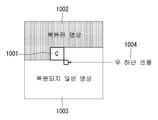•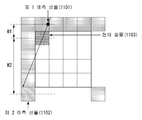•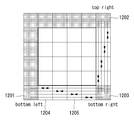•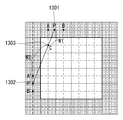•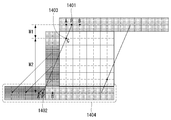•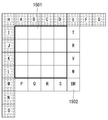•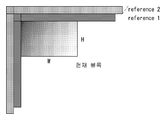••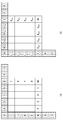•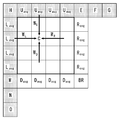•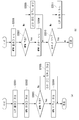•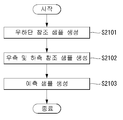•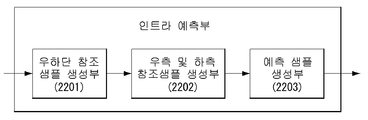•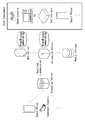## Classifications

• HELECTRICITY
• H04ELECTRIC COMMUNICATION TECHNIQUE
• H04NPICTORIAL COMMUNICATION, e.g. TELEVISION
• H04N19/00Methods or arrangements for coding, decoding, compressing or decompressing digital video signals
• H04N19/10Methods or arrangements for coding, decoding, compressing or decompressing digital video signals using adaptive coding
• H04N19/102Methods or arrangements for coding, decoding, compressing or decompressing digital video signals using adaptive coding characterised by the element, parameter or selection affected or controlled by the adaptive coding
• H04N19/103Selection of coding mode or of prediction mode
• H04N19/11Selection of coding mode or of prediction mode among a plurality of spatial predictive coding modes
• HELECTRICITY
• H04ELECTRIC COMMUNICATION TECHNIQUE
• H04NPICTORIAL COMMUNICATION, e.g. TELEVISION
• H04N19/00Methods or arrangements for coding, decoding, compressing or decompressing digital video signals
• H04N19/10Methods or arrangements for coding, decoding, compressing or decompressing digital video signals using adaptive coding
• H04N19/102Methods or arrangements for coding, decoding, compressing or decompressing digital video signals using adaptive coding characterised by the element, parameter or selection affected or controlled by the adaptive coding
• H04N19/132Sampling, masking or truncation of coding units, e.g. adaptive resampling, frame skipping, frame interpolation or high-frequency transform coefficient masking
• HELECTRICITY
• H04ELECTRIC COMMUNICATION TECHNIQUE
• H04NPICTORIAL COMMUNICATION, e.g. TELEVISION
• H04N19/00Methods or arrangements for coding, decoding, compressing or decompressing digital video signals
• H04N19/10Methods or arrangements for coding, decoding, compressing or decompressing digital video signals using adaptive coding
• H04N19/169Methods or arrangements for coding, decoding, compressing or decompressing digital video signals using adaptive coding characterised by the coding unit, i.e. the structural portion or semantic portion of the video signal being the object or the subject of the adaptive coding
• H04N19/17Methods or arrangements for coding, decoding, compressing or decompressing digital video signals using adaptive coding characterised by the coding unit, i.e. the structural portion or semantic portion of the video signal being the object or the subject of the adaptive coding the unit being an image region, e.g. an object
• H04N19/176Methods or arrangements for coding, decoding, compressing or decompressing digital video signals using adaptive coding characterised by the coding unit, i.e. the structural portion or semantic portion of the video signal being the object or the subject of the adaptive coding the unit being an image region, e.g. an object the region being a block, e.g. a macroblock
• HELECTRICITY
• H04ELECTRIC COMMUNICATION TECHNIQUE
• H04NPICTORIAL COMMUNICATION, e.g. TELEVISION
• H04N19/00Methods or arrangements for coding, decoding, compressing or decompressing digital video signals
• H04N19/50Methods or arrangements for coding, decoding, compressing or decompressing digital video signals using predictive coding
• H04N19/593Methods or arrangements for coding, decoding, compressing or decompressing digital video signals using predictive coding involving spatial prediction techniques

## Abstract

An image processing method based on an intra-prediction mode, and an associated apparatus. Specifically, the intra-prediction mode-based image processing method may comprise the steps of: generating a lower right reference sample adjacent to the lower right side of a current block; generate a right reference sample of the current block via the linear interpolation of a higher right reference sample and the lower right reference sample of the current block, and generate a lower reference sample of the current block via linear interpolation a lower left reference sample and the lower right reference sample of the current block; and generating a prediction sample of the current block using a left reference sample, an upper reference sample, a right reference sample, and a lower reference sample of the current block.

## Description

Intra prediction mode based image processing method and apparatus therefor

BACKGROUND OF THE INVENTION 1. Field of the Invention The present invention relates to a still image or moving image processing method, and more particularly, to a method of encoding / decoding a still image or moving image based on an intra prediction mode and an apparatus for supporting the same.

Compressive encoding refers to a series of signal processing techniques for transmitting digitized information over a communication line or for storing it in a form suitable for a storage medium. Media such as video, image, and audio can be subject to compression coding. In particular, a technique for performing compression coding on an image is referred to as video image compression.

Next-generation video content will feature high spatial resolution, high frame rate, and high dimensionality of scene representation. Processing such content will result in a tremendous increase in terms of memory storage, memory access rate, and processing power.

Therefore, there is a need to design a coding tool for processing next generation video contents more efficiently.

An object of the present invention is to provide a linear interpolation intra prediction method of generating a weighted prediction sample based on a distance between a current sample and a reference sample.

It is also an object of the present invention to provide a method of performing intra prediction using neighboring reference samples of a current block including reference samples of a previously unreversed region.

It is also an object of the present invention to provide a linear interpolation intra-DC prediction method using more reference samples than the conventional DC mode.

The technical objects to be achieved by the present invention are not limited to the above-mentioned technical problems, and other technical subjects which are not mentioned are described in the following description, which will be clearly understood by those skilled in the art to which the present invention belongs It will be possible.

According to an aspect of the present invention, there is provided a method of processing an image based on an intra prediction mode, the method comprising: generating a lower right reference sample adjacent to a lower right side of a current block; A left reference sample of the current block and a lower right reference sample are linearly interpolated by linearly interpolating the upper right reference sample and the lower right reference sample of the current block to linearly interpolate the lower left reference sample and the lower right reference sample, Generating a lower reference sample of the lower reference sample; And generating a prediction sample of the current block using a left reference sample, an upper reference sample, a right reference sample, and a lower reference sample of the current block.

Advantageously, the step of generating a prediction sample of the current block further comprises generating a prediction sample of the current block by averaging the left reference sample, the upper reference sample, the right reference sample and the lower reference samples can do.

Preferably, the step of generating a prediction sample of the current block includes averaging the samples of the left reference sample, the upper reference sample, the right reference sample, and the lower reference sample to calculate a left average value, an upper average value, Deriving an average value; And generating a prediction sample of the current block by linearly interpolating the left mean value, the upper mean value, the right mean value, and the lower mean value.

The generating of the prediction sample of the current block may include: calculating a weight according to a distance between a current pixel in the current block and a reference sample in each direction of the current block; And generating a prediction sample of the current pixel by applying the calculated weight to the left average value, the upper average value, the right average value, and the lower average value, respectively, to perform linear interpolation.

According to another aspect of the present invention, there is provided an apparatus for processing an image based on an intra prediction mode, the apparatus comprising: a lower right reference sample generation unit for generating a lower right reference sample adjacent to a lower right side of a current block; A left reference sample of the current block and a lower right reference sample are linearly interpolated by linearly interpolating the upper right reference sample and the lower right reference sample of the current block to linearly interpolate the lower left reference sample and the lower right reference sample, A right and a lower reference sample generator for generating a lower reference sample of the reference sample; And a prediction sample generator for generating a prediction sample of the current block using a left reference sample, an upper reference sample, a right reference sample, and a lower reference sample of the current block.

The prediction sample generator may generate a prediction sample of the current block by averaging the left reference sample, the upper reference sample, the right reference sample, and the lower reference sample.

Preferably, the prediction sample generator derives a left average value, an upper average value, a right average value, and a lower average value by averaging the samples of the left reference sample, the upper reference sample, the right reference sample, and the lower reference sample, The predicted sample of the current block may be generated by linearly interpolating the average value, the upper average value, the right mean value, and the lower mean value.

Preferably, the prediction sample generator calculates a weight according to a distance between a current pixel in the current block and a reference sample in each direction of the current block, and outputs the calculated weight to the left average value, the upper average value, And applying a linear interpolation to each of the lower average values to generate a prediction sample of the current pixel.

According to the embodiment of the present invention, it is possible to improve prediction accuracy by generating a plurality of prediction samples based on the intra prediction mode and linearly interpolating the generated prediction samples.

In addition, according to the embodiment of the present invention, prediction error can be reduced and compression performance can be improved by applying a weight according to the distance between the current sample and the reference sample in each direction.

The effects obtained in the present invention are not limited to the effects mentioned above, and other effects not mentioned can be clearly understood by those skilled in the art from the following description .

The accompanying drawings, which are included to provide a further understanding of the invention and are incorporated in and constitute a part of the specification, illustrate embodiments of the invention and, together with the description, serve to explain the technical features of the invention.

FIG. 1 is a schematic block diagram of an encoder in which still image or moving picture signal encoding is performed according to an embodiment of the present invention.

2 is a schematic block diagram of a decoder in which still image or moving picture signal encoding is performed according to an embodiment of the present invention.

3 is a diagram for explaining a division structure of a coding unit applicable to the present invention.

4 is a diagram for explaining a prediction unit that can be applied to the present invention.

5 is a diagram illustrating an intra prediction method according to an embodiment to which the present invention is applied.

FIG. 6 illustrates a prediction direction according to an intra prediction mode.

FIG. 7 is a diagram for explaining a linear interpolation prediction method, to which the present invention can be applied.

FIG. 8 illustrates region division according to the direction and angle of the intra-prediction mode.

9 is a diagram illustrating a method for generating a bottom-right sample in accordance with one embodiment of the present invention.

10 is a diagram illustrating a method of generating a bottom-right sample according to an embodiment of the present invention.

11 is a diagram for explaining a linear interpolation predicting method to which the present invention is applied.

12 is a view for explaining a method of generating a lower end sample and a right end sample according to an embodiment of the present invention.

FIG. 13 is a diagram for explaining a linear interpolation prediction method, to which the present invention is applied.

FIG. 14 is a diagram for explaining a linear interpolation prediction method, to which the present invention is applied.

15 is a diagram for explaining a linear interpolation prediction method according to an embodiment of the present invention.

16 is a diagram illustrating an intra prediction method using a multi-reference sample line as an embodiment to which the present invention is applied.

17 and 18 are diagrams for explaining a linear interpolation prediction method according to an embodiment of the present invention.

19 is a flowchart illustrating a linear interpolation prediction method according to an embodiment of the present invention.

FIG. 20 is a flowchart for explaining a syntax parsing routine for linear interpolation prediction, to which the present invention is applied.

21 is a diagram illustrating an intra prediction mode based linear interpolation prediction method according to an embodiment of the present invention.

22 is a diagram specifically illustrating an intra predictor according to an embodiment of the present invention.

FIG. 23 shows a structure of a content streaming system as an embodiment to which the present invention is applied.

Hereinafter, preferred embodiments according to the present invention will be described in detail with reference to the accompanying drawings. DETAILED DESCRIPTION OF THE PREFERRED EMBODIMENTS The following detailed description, together with the accompanying drawings, is intended to illustrate exemplary embodiments of the invention and is not intended to represent the only embodiments in which the invention may be practiced. The following detailed description includes specific details in order to provide a thorough understanding of the present invention. However, those skilled in the art will appreciate that the present invention may be practiced without these specific details.

In some instances, well-known structures and devices may be omitted or may be shown in block diagram form, centering on the core functionality of each structure and device, to avoid obscuring the concepts of the present invention.

In addition, although the term used in the present invention is selected as a general term that is widely used as far as possible, a specific term will be described using a term arbitrarily selected by the applicant. In such a case, the meaning is clearly stated in the detailed description of the relevant part, so it should be understood that the name of the term used in the description of the present invention should not be simply interpreted and that the meaning of the corresponding term should be understood and interpreted .

The specific terminology used in the following description is provided to aid understanding of the present invention, and the use of such specific terminology may be changed into other forms without departing from the technical idea of the present invention. For example, signals, data, samples, pictures, frames, blocks, etc. may be appropriately replaced in each coding process.

Herein, 'processing unit' means a unit in which processing of encoding / decoding such as prediction, conversion and / or quantization is performed. Hereinafter, the processing unit may be referred to as a &quot; processing block &quot; or a &quot; block &quot;

The processing unit may be interpreted to include a unit for the luma component and a unit for the chroma component. For example, the processing unit may correspond to a coding tree unit (CTU), a coding unit (CU), a prediction unit (PU), or a transform unit (TU).

Further, the processing unit can be interpreted as a unit for a luminance (luma) component or as a unit for a chroma component. For example, the processing unit may include a Coding Tree Block (CTB), a Coding Block (CB), a Prediction Block (PU), or a Transform Block (TB) ). Or may correspond to a coding tree block (CTB), a coding block (CB), a prediction block (PU) or a transform block (TB) for a chroma component. Also, the present invention is not limited to this, and the processing unit may be interpreted to include a unit for the luma component and a unit for the chroma component.

Further, the processing unit is not necessarily limited to a square block, but may be configured as a polygonal shape having three or more vertexes.

In the following description, a pixel, a pixel, or the like is collectively referred to as a sample. And, using a sample may mean using a pixel value, a pixel value, or the like.

FIG. 1 is a schematic block diagram of an encoder in which still image or moving picture signal encoding is performed according to an embodiment of the present invention.

1, an encoder 100 includes an image divider 110, a subtractor 115, a transformer 120, a quantizer 130, an inverse quantizer 140, an inverse transformer 150, A decoding unit 160, a decoded picture buffer (DPB) 170, a predicting unit 180, and an entropy encoding unit 190. The prediction unit 180 may include an inter prediction unit 181 and an intra prediction unit 182.

The image divider 110 divides an input video signal (or a picture, a frame) input to the encoder 100 into one or more processing units.

The subtractor 115 subtracts a prediction signal (or a prediction block) output from the prediction unit 180 (i.e., the inter prediction unit 181 or the intra prediction unit 182) from the input video signal, And generates a residual signal (or difference block). The generated difference signal (or difference block) is transmitted to the conversion unit 120.

The transforming unit 120 transforms a difference signal (or a difference block) by a transform technique (for example, DCT (Discrete Cosine Transform), DST (Discrete Sine Transform), GBT (Graph-Based Transform), KLT (Karhunen- Etc.) to generate a transform coefficient. At this time, the transform unit 120 may generate transform coefficients by performing transform using a transform technique determined according to a prediction mode applied to a difference block and a size of a difference block.

The quantization unit 130 quantizes the transform coefficients and transmits the quantized transform coefficients to the entropy encoding unit 190. The entropy encoding unit 190 entropy-codes the quantized signals and outputs them as a bitstream.

Meanwhile, the quantized signal output from the quantization unit 130 may be used to generate a prediction signal. For example, the quantized signal can be reconstructed by applying inverse quantization and inverse transformation through the inverse quantization unit 140 and the inverse transform unit 150 in the loop. A reconstructed signal can be generated by adding the reconstructed difference signal to a prediction signal output from the inter prediction unit 181 or the intra prediction unit 182. [

On the other hand, in the compression process as described above, adjacent blocks are quantized by different quantization parameters, so that deterioration of the block boundary can be generated. This phenomenon is called blocking artifacts, and this is one of the important factors for evaluating image quality. A filtering process can be performed to reduce such deterioration. Through the filtering process, blocking deterioration is eliminated and the error of the current picture is reduced, thereby improving the image quality.

The filtering unit 160 applies filtering to the restored signal and outputs the restored signal to the playback apparatus or the decoded picture buffer 170. The filtered signal transmitted to the decoding picture buffer 170 may be used as a reference picture in the inter-prediction unit 181. [ As described above, not only the picture quality but also the coding efficiency can be improved by using the filtered picture as a reference picture in the inter picture prediction mode.

The decoded picture buffer 170 may store the filtered picture for use as a reference picture in the inter-prediction unit 181. [

The inter-prediction unit 181 performs temporal prediction and / or spatial prediction to remove temporal redundancy and / or spatial redundancy with reference to a reconstructed picture. Here, since the reference picture used for prediction is a transformed signal obtained through quantization and inverse quantization in units of blocks at the time of encoding / decoding in the previous time, blocking artifacts or ringing artifacts may exist have.

Accordingly, the inter-prediction unit 181 can interpolate signals between pixels by sub-pixel by applying a low-pass filter in order to solve the performance degradation due to discontinuity or quantization of such signals. Here, a subpixel means a virtual pixel generated by applying an interpolation filter, and an integer pixel means an actual pixel existing in a reconstructed picture. As the interpolation method, linear interpolation, bi-linear interpolation, wiener filter and the like can be applied.

The interpolation filter may be applied to a reconstructed picture to improve the accuracy of the prediction. For example, the inter-prediction unit 181 generates an interpolation pixel by applying an interpolation filter to an integer pixel, and uses an interpolated block composed of interpolated pixels as a prediction block Prediction can be performed.

The intra predictor 182 predicts a current block by referring to samples in the vicinity of a block to be currently encoded. The intraprediction unit 182 may perform the following procedure to perform intra prediction. First, a reference sample necessary for generating a prediction signal can be prepared. Then, a prediction signal can be generated using the prepared reference sample. Thereafter, the prediction mode is encoded. At this time, reference samples can be prepared through reference sample padding and / or reference sample filtering. Since the reference samples have undergone prediction and reconstruction processes, quantization errors may exist. Therefore, a reference sample filtering process can be performed for each prediction mode used for intraprediction to reduce such errors.

In particular, the intra predictor 182 according to the present invention can perform intra prediction on a current block by linearly interpolating prediction sample values generated based on an intra prediction mode of the current block. A more detailed description of the intra predictor 182 will be described later.

A prediction signal (or a prediction block) generated through the inter prediction unit 181 or the intra prediction unit 182 is used to generate a reconstruction signal (or reconstruction block) or a difference signal (or a difference block) / RTI &gt;

2 is a schematic block diagram of a decoder in which still image or moving picture signal encoding is performed according to an embodiment of the present invention.

2, the decoder 200 includes an entropy decoding unit 210, an inverse quantization unit 220, an inverse transform unit 230, an adder 235, a filtering unit 240, a decoded picture buffer (DPB) A buffer unit 250, and a prediction unit 260. The prediction unit 260 may include an inter prediction unit 261 and an intra prediction unit 262.

The reconstructed video signal output through the decoder 200 may be reproduced through a reproducing apparatus.

The decoder 200 receives a signal (i.e., a bit stream) output from the encoder 100 of FIG. 1, and the received signal is entropy-decoded through the entropy decoding unit 210.

The inverse quantization unit 220 obtains a transform coefficient from the entropy-decoded signal using the quantization step size information.

The inverse transform unit 230 obtains a residual signal (or a difference block) by inverse transforming the transform coefficient by applying an inverse transform technique.

The adder 235 adds the obtained difference signal (or difference block) to the prediction signal output from the prediction unit 260 (i.e., the inter prediction unit 261 or the intra prediction unit 262) ) To generate a reconstructed signal (or reconstruction block).

The filtering unit 240 applies filtering to a reconstructed signal (or a reconstructed block) and outputs it to a reproducing apparatus or transmits the reconstructed signal to a decoding picture buffer unit 250. The filtered signal transmitted to the decoding picture buffer unit 250 may be used as a reference picture in the inter prediction unit 261.

The embodiments described in the filtering unit 160, the inter-prediction unit 181 and the intra-prediction unit 182 of the encoder 100 respectively include the filtering unit 240 of the decoder, the inter-prediction unit 261, The same can be applied to the intra prediction unit 262.

In particular, the intra-prediction unit 262 according to the present invention can perform intra-prediction on a current block by linearly interpolating prediction sample values generated based on an intra-prediction mode of the current block. A more detailed description of the intra prediction unit 262 will be described later.

Generally, a block-based image compression method is used in a still image or moving image compression technique (for example, HEVC). A block-based image compression method is a method of dividing an image into a specific block unit, and can reduce memory usage and computation amount.

3 is a diagram for explaining a division structure of a coding unit applicable to the present invention.

The encoder divides one image (or picture) into units of a rectangular shaped coding tree unit (CTU: Coding Tree Unit). Then, one CTU is sequentially encoded according to a raster scan order.

In HEVC, the size of CTU can be set to 64 × 64, 32 × 32, or 16 × 16. The encoder can select the size of the CTU according to the resolution of the input image or characteristics of the input image. The CTU includes a coding tree block (CTB) for a luma component and a CTB for two chroma components corresponding thereto.

One CTU can be partitioned into a quad-tree structure. That is, one CTU is divided into four units having a square shape and having a half horizontal size and a half vertical size to generate a coding unit (CU) have. This division of the quad-tree structure can be performed recursively. That is, the CU is hierarchically partitioned from one CTU to a quad-tree structure.

The CU means a basic unit of coding in which processing of an input image, for example, intra / inter prediction is performed. The CU includes a coding block (CB) for the luma component and CB for the corresponding two chroma components. In HEVC, the size of CU can be set to 64 × 64, 32 × 32, 16 × 16, or 8 × 8.

Referring to FIG. 3, the root node of the quad-tree is associated with the CTU. The quad-tree is divided until it reaches the leaf node, and the leaf node corresponds to the CU.

More specifically, the CTU corresponds to a root node and has the smallest depth (i.e., depth = 0). Depending on the characteristics of the input image, the CTU may not be divided. In this case, the CTU corresponds to the CU.

The CTU can be partitioned into a quad tree form, resulting in subnodes with depth 1 (depth = 1). A node that is not further divided in the lower node having a depth of 1 (i.e., leaf node) corresponds to a CU. For example, CU (a), CU (b), and CU (j) corresponding to nodes a, b, and j in FIG. 3B are divided once in the CTU and have a depth of one.

At least one of the nodes having a depth of 1 can be further divided into a quadtree form, so that the lower nodes having a depth 1 (i.e., depth = 2) are generated. A node that is not further divided in the lower node having a depth of 2 (i.e., a leaf node) corresponds to a CU. For example, CU (c), CU (h) and CU (i) corresponding to nodes c, h and i in FIG. 3B are divided twice in the CTU and have a depth of 2.

Also, at least one of the nodes having a depth of 2 can be further divided into a quad tree form, so that the lower nodes having a depth of 3 (i.e., depth = 3) are generated. A node that is not further divided in the lower node having a depth of 3 corresponds to a CU. For example, CU (d), CU (e), CU (f) and CU (g) corresponding to nodes d, e, f and g in FIG. Depth.

In the encoder, the maximum size or the minimum size of the CU can be determined according to the characteristics of the video image (for example, resolution) or considering the efficiency of encoding. Information on this or information capable of deriving the information may be included in the bitstream. A CU having a maximum size is called a Largest Coding Unit (LCU), and a CU having a minimum size can be referred to as a Smallest Coding Unit (SCU).

Also, a CU having a tree structure can be hierarchically divided with a predetermined maximum depth information (or maximum level information). Each divided CU can have depth information. The depth information indicates the number and / or degree of division of the CU, and therefore may include information on the size of the CU.

Since the LCU is divided into quad tree form, the size of the SCU can be obtained by using the LCU size and the maximum depth information. Conversely, by using the size of the SCU and the maximum depth information of the tree, the size of the LCU can be obtained.

For one CU, information indicating whether the corresponding CU is divided (for example, a split CU flag (split_cu_flag)) may be transmitted to the decoder. This partitioning information is included in all CUs except SCU. For example, if the value of the flag indicating division is '1', the corresponding CU is again divided into four CUs. If the flag indicating the division is '0', the corresponding CU is not further divided, Can be performed.

As described above, the CU is a basic unit of coding in which intra prediction or inter prediction is performed. The HEVC divides the CU into units of Prediction Unit (PU) in order to more effectively code the input image.

PU is a basic unit for generating prediction blocks, and it is possible to generate prediction blocks in units of PU different from each other in a single CU. However, PUs belonging to one CU are not mixed with intra prediction and inter prediction, and PUs belonging to one CU are coded by the same prediction method (i.e., intra prediction or inter prediction).

The PU is not divided into a quad-tree structure, and is divided into a predetermined form in one CU. This will be described with reference to the following drawings.

4 is a diagram for explaining a prediction unit that can be applied to the present invention.

The PU is divided according to whether the intra prediction mode is used or the inter prediction mode is used in the coding mode of the CU to which the PU belongs.

FIG. 4A illustrates a PU when an intra prediction mode is used, and FIG. 4B illustrates a PU when an inter prediction mode is used.

Referring to FIG. 4A, assuming that the size of one CU is 2N × 2N (N = 4, 8, 16, and 32), one CU has two types (ie, 2N × 2N or N X N).

Here, when divided into 2N × 2N type PUs, it means that only one PU exists in one CU.

On the other hand, in case of dividing into N × N type PUs, one CU is divided into four PUs, and different prediction blocks are generated for each PU unit. However, the division of the PU can be performed only when the size of the CB with respect to the luminance component of the CU is the minimum size (i.e., when the CU is the SCU).

Referring to FIG. 4B, assuming that the size of one CU is 2N × 2N (N = 4, 8, 16, and 32), one CU has eight PU types (ie, 2N × 2N , NN, 2NN, NNN, NLNN, NRNN, 2NNU, 2NND).

Similar to intraprediction, N × N type PU segmentation can be performed only when the size of the CB for the luminance component of the CU is the minimum size (ie, when the CU is SCU).

In the inter prediction, 2N × N type division in the horizontal direction and N × 2N type PU division in the vertical direction are supported.

In addition, it supports PU segmentation of nL × 2N, nR × 2N, 2N × nU, and 2N × nD types in the form of Asymmetric Motion Partition (AMP). Here, 'n' means a 1/4 value of 2N. However, the AMP can not be used when the CU to which the PU belongs is the minimum size CU.

The optimal division structure of the coding unit (CU), the prediction unit (PU), and the conversion unit (TU) for efficiently encoding an input image in one CTU is a rate-distortion- Value. &Lt; / RTI &gt; For example, if we look at the optimal CU partitioning process within a 64 × 64 CTU, the rate-distortion cost can be calculated by dividing from a 64 × 64 CU to an 8 × 8 CU. The concrete procedure is as follows.

1) Determine the optimal PU and TU partition structure that generates the minimum rate-distortion value through inter / intra prediction, transform / quantization, dequantization / inverse transformation, and entropy encoding for 64 × 64 CUs.

2) Divide the 64 × 64 CU into 4 32 × 32 CUs and determine the partition structure of the optimal PU and TU to generate the minimum rate-distortion value for each 32 × 32 CU.

3) 32 × 32 CUs are subdivided into 4 16 × 16 CUs to determine the optimal PU and TU partition structure that yields the minimum rate-distortion value for each 16 × 16 CU.

4) Divide the 16 × 16 CU into 4 8 × 8 CUs and determine the optimal PU and TU partition structure that yields the minimum rate-distortion value for each 8 × 8 CU.

5) The sum of the 16 × 16 CU rate-distortion values calculated in the above procedure 3) and the sum of the 4 8 × 8 CU rate-distortion values calculated in the process 4) Lt; RTI ID = 0.0 &gt; CU &lt; / RTI &gt; This process is also performed for the remaining three 16 × 16 CUs.

6) The sum of the 32 × 32 CU rate-distortion values calculated in the process 2) above and the sum of the 4 16 × 16 CU rate-distortion values obtained in the process 5) Lt; RTI ID = 0.0 &gt; CU &lt; / RTI &gt; This process is also performed for the remaining three 32 × 32 CUs.

7) Finally, we compare the sum of the rate-distortion values of 64 × 64 CUs calculated in the process of the above 1) and the rate-distortion values of the four 32 × 32 CUs obtained in the process of the above 6) The optimal CU division structure is determined within the x 64 blocks.

In the intra prediction mode, a prediction mode is selected in units of PU, and prediction and reconstruction are performed in units of actual TUs for the selected prediction mode.

TU means the basic unit on which the actual prediction and reconstruction are performed. The TU includes a transform block (TB) for the luma component and a TB for the two chroma components corresponding thereto.

In the example of FIG. 3, the TU is hierarchically divided into a quad-tree structure from one CU to be coded, as one CTU is divided into a quad-tree structure to generate a CU.

Since the TU is divided into quad-tree structures, the TUs segmented from the CUs can be further divided into smaller lower TUs. In HEVC, the size of the TU can be set to any one of 32 × 32, 16 × 16, 8 × 8, and 4 × 4.

Referring again to FIG. 3, it is assumed that the root node of the quadtree is associated with a CU. The quad-tree is divided until it reaches a leaf node, and the leaf node corresponds to TU.

More specifically, the CU corresponds to a root node and has the smallest depth (i.e., depth = 0). Depending on the characteristics of the input image, the CU may not be divided. In this case, the CU corresponds to the TU.

The CU can be partitioned into a quadtree form, resulting in sub-nodes with depth 1 (depth = 1). Then, a node that is not further divided in the lower node having a depth of 1 (i.e., leaf node) corresponds to TU. For example, TU (a), TU (b), and TU (j) corresponding to nodes a, b, and j in FIG. 3B are once partitioned in the CU and have a depth of one.

At least one of the nodes having a depth of 1 can be further divided into a quadtree form, so that the lower nodes having a depth 1 (i.e., depth = 2) are generated. And, the node that is not further divided in the lower node having the depth of 2 (ie leaf node) corresponds to TU. For example, TU (c), TU (h) and TU (i) corresponding to nodes c, h and i in FIG. 3B are divided twice in CU and have a depth of 2.

Also, at least one of the nodes having a depth of 2 can be further divided into a quad tree form, so that the lower nodes having a depth of 3 (i.e., depth = 3) are generated. A node that is not further divided in the lower node having a depth of 3 corresponds to a CU. For example, TU (d), TU (e), TU (f), and TU (g) corresponding to nodes d, e, f and g in FIG. Depth.

A TU having a tree structure can be hierarchically divided with predetermined maximum depth information (or maximum level information). Then, each divided TU can have depth information. The depth information indicates the number and / or degree of division of the TU, and therefore may include information on the size of the TU.

For one TU, information indicating whether the corresponding TU is divided (e.g., a split TU flag (split_transform_flag)) may be communicated to the decoder. This partitioning information is included in all TUs except the minimum size TU. For example, if the value of the flag indicating whether or not to divide is '1', the corresponding TU is again divided into four TUs, and if the flag indicating the division is '0', the corresponding TU is no longer divided.

Prediction

And may use the decoded portion of the current picture or other pictures that contain the current processing unit to recover the current processing unit in which decoding is performed.

A picture (slice) that uses only the current picture, that is, a picture (slice) that uses only the current picture, that is, a picture (slice) that performs only intra-picture prediction is referred to as an intra picture or an I picture A picture (slice) using a predictive picture or a P picture (slice), a maximum of two motion vectors and a reference index may be referred to as a bi-predictive picture or a B picture (slice).

Intra prediction refers to a prediction method that derives the current processing block from a data element (e.g., a sample value, etc.) of the same decoded picture (or slice). That is, it means a method of predicting the pixel value of the current processing block by referring to the reconstructed areas in the current picture.

Inter prediction refers to a prediction method of deriving a current processing block based on a data element (e.g., a sample value or a motion vector) of a picture other than the current picture. That is, this means a method of predicting pixel values of a current processing block by referring to reconstructed areas in other reconstructed pictures other than the current picture.

Hereinafter, intra prediction (or intra prediction) will be described in more detail.

Intra  prediction( Intra  prediction (or intra prediction)

5 is a diagram illustrating an intra prediction method according to an embodiment to which the present invention is applied.

Referring to FIG. 5, the decoder derives an intra prediction mode of the current processing block (S501).

In intra prediction, it is possible to have a prediction direction with respect to the position of a reference sample used for prediction according to the prediction mode. An intra prediction mode having a prediction direction is referred to as an intra prediction mode (Intra_Angular prediction mode). On the other hand, there are an intra-planar (INTRA_PLANAR) prediction mode and an intra-DC (INTRA_DC) prediction mode as intra-prediction modes having no prediction direction.

Table 1 illustrates the intra-prediction mode and related names, and FIG. 6 illustrates the prediction direction according to the intra-prediction mode.

In intra prediction, prediction is performed on the current processing block based on the derived prediction mode. Since the reference sample used in the prediction differs from the concrete prediction method used in the prediction mode according to the prediction mode, when the current block is encoded in the intra prediction mode, the decoder derives the prediction mode of the current block in order to perform prediction.

The decoder checks whether neighboring samples of the current processing block can be used for prediction, and constructs reference samples to be used for prediction (S502).

In the intra prediction, neighbor samples of the current processing block include a sample adjacent to the left boundary of the current processing block of size nS x nS and a total of 2 x nS samples neighboring the bottom-left, A sample adjacent to the top boundary and a total of 2 x n S samples neighboring the top-right side and one sample neighboring the top-left of the current processing block.

However, some of the surrounding samples of the current processing block may not yet be decoded or may not be available. In this case, the decoder may substitute samples that are not available with the available samples to construct reference samples for use in prediction.

The decoder may perform filtering of the reference samples based on the intra prediction mode (S503).

Whether or not the filtering of the reference sample is performed can be determined based on the size of the current processing block. In addition, the filtering method of the reference sample may be determined by a filtering flag transmitted from the encoder.

The decoder generates a prediction block for the current processing block based on the intra prediction mode and the reference samples (S504). That is, the decoder determines the intra prediction mode derived in the intra prediction mode deriving step S501, the prediction for the current processing block based on the reference samples acquired in the reference sample building step S502 and the reference sample filtering step S503, (I.e., generates a prediction sample).

In order to minimize the discontinuity of the boundary between the processing blocks when the current processing block is encoded in the INTRA_DC mode, the left boundary sample of the prediction block (i.e., the sample in the prediction block adjacent to the left boundary) (that is, samples in the prediction block adjacent to the upper boundary).

Also, in step S504, filtering may be applied to the left boundary sample or the upper boundary sample, similar to the INTRA_DC mode, for the vertical direction mode and the horizontal direction mode of the intra directional prediction modes.

More specifically, when the current processing block is encoded in a vertical mode or a horizontal mode, the value of a predicted sample can be derived based on a reference sample located in a prediction direction. At this time, among the left boundary sample or the upper boundary sample of the prediction block, the boundary sample which is not located in the prediction direction may be adjacent to the reference sample which is not used for prediction. That is, the distance from the reference sample that is not used for prediction may be much closer than the distance from the reference sample used for prediction.

Accordingly, the decoder may adaptively apply filtering to the left boundary samples or the upper boundary samples according to whether the intra-prediction direction is vertical or horizontal. That is, when the intra prediction direction is vertical, filtering is applied to the left boundary samples, and filtering is applied to the upper boundary samples when the intra prediction direction is the horizontal direction.

As described above, HEVC generates prediction blocks using 33 directional prediction methods, two non-directional prediction methods, and 35 prediction methods through intraprediction (or intra prediction). The conventional intra prediction method generates a prediction sample using a surrounding reference sample (assuming that it is encoded / decoded in a raster scan order, an upper reference sample or a left reference sample). Then, the generated prediction sample is copied to the prediction sample generated according to the direction of the intra prediction mode.

Since the predicted sample value is simply copied in accordance with the prediction direction, there arises a problem that the accuracy of the prediction decreases as the distance from the reference sample increases. That is, if the distance between the reference samples used for prediction and the prediction sample is close, the prediction accuracy is high. However, if the distance between the reference sample used for prediction and the prediction sample is far, the prediction accuracy is low.

In order to solve the above problems, the present invention proposes a linear interpolation intra prediction method applying a weight according to a distance between a current sample and a reference sample. In an embodiment of the present invention, the encoder / decoder may derive a plurality of prediction samples using peripheral reference samples and linearly interpolate the derived prediction samples to produce a final prediction sample. Hereinafter, the neighboring samples of the invention proposed herein may refer to samples available in intra prediction. In the description of the present invention, neighboring samples will be described below for convenience of explanation, but the present invention is not limited thereto.

Neighboring samples include a sample adjacent to the left boundary of the current block of size nS 占 nS and a total of 2 占 n Samples neighboring the bottom-left, a sample adjacent to the top boundary of the current block And a total of 2 x n S samples neighboring the top-right and one sample neighboring the top-left of the current block. The neighboring samples may also include nS samples adjacent to the right boundary of the current block, nS samples adjacent to the bottom boundary of the current block, and bottom-right samples of the current block, Lt; RTI ID = 0.0 &gt; 1). &Lt; / RTI &gt;

In the description of the present invention, for convenience of explanation, a sample adjacent to the top-right and a sample adjacent to the bottom-left are described as follows, but the present invention is not limited thereto. The samples neighboring the top-right of the current block are [nS, -1] to [2 * nS-1], assuming that the horizontal and vertical coordinates of the upper left sample of the current block are [0,0] , -1], respectively. Also, a sample neighboring the bottom-left of the current block may mean nS samples located at [-1, nS] - [-1, 2 * nS-1].

The linear interpolation intra prediction method derives a first predicted sample value and a second predicted sample value using neighboring samples of a current block, linearly interpolates the derived first predicted sample value and second predicted sample value, Can be generated. After dividing the intra prediction mode into regions, one reference sample may be determined as a reference sample for linear interpolation on a block-by-block basis to derive a second predicted sample value, or a sample may be generated on the basis of the direction and angle of the intra- The second predicted sample value may be derived from one of the neighboring samples and the second predicted sample value may be derived from the linear interpolation between the two reference samples.

First, a description will be given of a linear interpolation intra prediction method in which a reference sample for linear interpolation is determined as a reference sample for each block, and the second predicted sample value is derived from the determined reference sample value, after dividing the intra prediction mode into regions do. The encoder / decoder may derive the first predicted sample value and the second predicted sample value, and linearly interpolate the derived first predicted sample value and the derived second predicted sample value to generate a predicted sample of the current block.

The first predicted sample value may be derived by the method described in FIGS. 5 and 6. FIG. That is, the encoder / decoder derives the intra prediction mode of the current block, verifies that neighboring samples of the current block can be used for prediction, and constructs reference samples used for prediction.

For example, in the case of the HEVC, neighboring samples of the current block include samples adjacent to the left boundary of the current processing block of size nS 占 nS and neighboring samples at the bottom- 2 x n S samples, a sample adjacent to the top boundary of the current processing block, and a total of 2 x n S samples neighboring the top-right side and the top-left side of the current processing block It can mean one neighboring sample.

If some of the neighboring samples of the current block have not yet been decoded or are not available, the encoder / decoder may substitute samples that are not available with the available samples, And perform filtering of the reference samples based on the intra prediction mode. The encoder / decoder may derive the first predicted sample value using the intra prediction mode and the reference samples. The encoder / decoder may determine a reference sample for linear interpolation based on the direction and angle of the intra-prediction mode and determine a second predicted sample value with the determined reference sample value. A method of determining a reference sample for linear interpolation will be described below with reference to FIGS. 7 and 8. FIG.

FIG. 7 is a diagram for explaining a linear interpolation prediction method, to which the present invention can be applied.

The encoder / decoder can determine different reference samples as reference samples for linear interpolation depending on the direction and angle of the intra prediction mode. And the encoder / decoder may use the reference sample value for the determined linear interpolation as the second predicted sample value. In the case of Fig. 7 (a), the reference sample for linear interpolation may be determined as the upper-right sample 701 adjacent to the current block.

7A, the encoder / decoder can linearly interpolate the first predicted sample value and the value of the upper-right sample 701 adjacent to the current block to generate a predicted sample value of the current sample. The encoder / decoder can generate a prediction block of the current block by applying the same method to all samples in the current block.

In Fig. 7 (b), the reference sample for linear interpolation may be determined as the lower-right sample 702 adjacent to the current block. In this case, the encoder / decoder may linearly interpolate the first predicted sample value and the lower-right sample 702 value adjacent to the current block to generate a predicted sample value of the current sample. The encoder / decoder can generate a prediction block of the current block by applying the same method to all samples in the current block.

7 (c), the reference sample for linear interpolation can be determined as the lower left sample 703 adjacent to the current block. In this case, the encoder / decoder can linearly interpolate the first predicted sample value and the lower-left sample 703 value adjacent to the current block to produce a predicted sample value of the current sample. The encoder / decoder can generate a prediction block of the current block by applying the same method to all samples in the current block.

That is, a reference sample for linear interpolation according to the direction and angle of the intra-prediction mode may be determined as the upper-left sample 701, the lower-right sample 702, or the lower left sample 703 adjacent to the current block. The determined reference sample value may be derived as a second predicted sample value.

As described above, the second predicted sample value may be derived using different reference samples depending on the direction and angle of the intra-prediction mode. Hereinafter, the direction and angle will be described with reference to the following drawings.

FIG. 8 illustrates region division according to the direction and angle of the intra-prediction mode.

Referring to FIG. 8, regions having horizontal directionality can be divided into regions A and B, and regions having vertical directionality can be divided into regions C and D. In addition, positive angular regions can be divided into A and D regions, and negative angular regions can be divided into B and C regions based on angles. Table 2 summarizes the division of regions according to the direction and angle of the prediction mode.

That is, the area A is a case where the direction of the intra-prediction mode is the horizontal direction and belongs to the area of the positive angular direction. The B region is a case where the direction of the intra-prediction mode is the horizontal direction and belongs to the region of the negative angular direction. The C region is a case in which the direction of the intra-prediction mode is a vertical direction and belongs to a region in the negative angular direction. The D region is a case in which the direction of the intra-prediction mode is a vertical direction and belongs to a region in a positive angular direction.

Referring to FIG. 7, FIG. 7A shows the case where the direction of the intra-prediction mode belongs to the A region, FIG. 7B shows the case of belonging to the B and C regions, .

That is, if the direction of the intra-prediction mode belongs to the A region (region in the horizontal direction and positive angular direction), the second predicted sample value can be derived as the value of the upper-right sample 701 adjacent to the current block.

When the direction of the intra prediction mode belongs to the B region (region in the horizontal direction and negative angular direction) or C region (region in the vertical direction and negative angular direction), the second predicted sample value is the lower- (702). &Lt; / RTI &gt;

If the direction of the intra prediction mode belongs to the D region (region of the vertical direction and positive angular direction), the second predicted sample value may be derived as the value of the lower left sample 703 adjacent to the current block.

In the case of the horizontal mode, the value of the upper right sample 701 adjacent to the current block may be derived as the second predicted sample value, and the value of the lower right sample 702 adjacent to the current block may be calculated as the second predicted sample value . In addition, a linear interpolation value (or averaged value) of the upper-left sample 701 and the lower-right sample 702 may be derived as the second predicted sample value.

In the case of the vertical mode, the value of the lower left sample 703 adjacent to the current block may be derived as the second predicted sample value, and the value of the lower right sample 702 adjacent to the current block may be derived as the second predicted sample value . &Lt; / RTI &gt; In addition, a linear interpolation (or averaged value) of the lower-left sample 703 and the lower-right sample 702 may be derived as a second predicted sample value.

B, and C, the lower right sample value adjacent to the current block can be derived as the second predicted sample value. At this time, the encoder / decoder may generate the bottom right sample to derive the second predicted sample value. A method of generating a lower-left sample (or a lower right-hand reference sample) with reference to the following drawings will be described.

9 is a diagram illustrating a method for generating a bottom-right sample in accordance with one embodiment of the present invention.

Referring to FIG. 9A, a lower right sample 901 adjacent to a current block and a lower left sample 902 adjacent to a current block can be used to generate a lower right sample 903 adjacent to a current block.

For example, the lower-right sample 901 and the lower-left sample 902 may be generated as the average value of the upper-left sample 901 and the lower-left sample 902, respectively. At this time, a lower right sample 903 may be generated using Equation (1).

Referring to FIG. 9 (b), a sample located at the rightmost side of a sample adjacent to the upper right end of the current block (hereinafter, referred to as an upper rightmost sample) (2 * nS-1, -1) samples (904) in a sample that is twice as long as the current block in the direction of the current block, i.e., in the nS x nS block, and a sample located at the lowermost (Hereinafter, referred to as an &quot; most left bottom sample &quot;) (e.g., a sample that is twice as long as the current block in the vertical direction on the basis of a sample adjacent to the upper left end of the current block, * nS-1] samples) 905 can be used to generate lower-right samples 906 adjacent to the current block.

For example, the value of the bottom right sample (906) can be generated by averaging the values of the right most upper left sample (904) and the most left lower right sample (905). At this time, the lower right sample 906 may be generated using Equation (2).

In FIG. 9, a method of generating lower-right samples using neighboring samples belonging to the reconstructed image by performing encoding / decoding has been described. A method of directly using a sample value of an original image will be described with reference to the following drawings.

10 is a diagram illustrating a method of generating a bottom-right sample according to an embodiment of the present invention.

Referring to FIG. 10, for example, when encoding / decoding is performed in the raster scan order, encoding / decoding is performed based on the current block C 1001 and the reconstructed image part 1002 is still encoded / (Or a portion of the image not restored) 1003, as shown in FIG. That is, the non-reconstructed image portion 1003, which is the right side and the lower side of the current block C 1001, may correspond to a portion where encoding / decoding has not yet been performed.

Since the lower-right sample 1004 adjacent to the current block C 1001 corresponds to a portion that has not yet been reconstructed, the decoder can not immediately use the lower-most sample 1004.

In this case, the encoder transmits a sample value corresponding to the lower-right sample 1004 adjacent to the current block C 1001 in the original image to the decoder, and the decoder receives the sample value and outputs the lower- The value can be used as is.

That is, the encoder can perform encoding using the value of the lower right sample (1004) of the original image, and the decoder can perform decoding using the value of the lower right sample (1004) received as it is.

Since the encoder / decoder uses the sampled values of the original image as it is, the prediction accuracy and compression performance can be improved.

The method of deriving the first predicted sample value and the second predicted sample value and the method of generating the lower-order sample have been described above. Hereinafter, a method of generating a predicted sample (or a final predicted sample) value of a current block by linearly interpolating a first predicted sample value and a second predicted sample value will be described with reference to the following drawings.

11 is a diagram for explaining a linear interpolation predicting method to which the present invention is applied.

In FIG. 11, for convenience of description, the case where the direction of the intra-prediction mode of the current block belongs to the area of the vertical direction and the positive angle direction (that is, the area D in FIG. 8) will be described as an example.

The encoder / decoder can derive the intra prediction mode of the current block, verify that neighboring samples can be used, and construct reference samples used for prediction. The encoder / decoder may derive the first predicted sample 1101 value using the derived intra prediction mode and reference samples.

As described above, when the direction of the intra-prediction mode of the current block is the vertical direction and belongs to the area in the positive angular direction, the value of the second predicted sample 1102 may be determined as the lower left sample value adjacent to the current block.

The encoder / decoder may linearly interpolate the value of the first predicted sample 1101 and the value of the second predicted sample 1102 to generate a predicted sample (or a final predicted sample) of the current sample 1103. At this time, the predicted sample value (or the final predicted sample) of the current sample 1103 can be calculated as shown in Equation (3).

In Equation (3), w1 is a vertical distance between the first predicted sample 1101 and the current sample 1103, and w2 is a vertical distance between the second predicted sample 1102 and the current sample 1103. [ That is, the encoder / decoder performs a linear interpolation by applying a weight according to the vertical distance ratio to the value of the first predicted sample 1101 and the value of the second predicted sample 1102, thereby generating a predicted sample of the current sample 1103 .

The encoder / decoder can generate a prediction block of the current block by applying the same method to all samples in the current block.

For a direction other than the direction of the intra prediction mode illustrated in FIG. 11, the predicted sample value of the current sample can be calculated using Equation (3).

That is, in all intraprediction modes having directionality (for example, an intra prediction mode with 33 directions in HEVC), the encoder / decoder calculates the vertical distance between the first predicted sample and the current sample, the vertical distance between the second predicted sample and the current sample The weighting according to the vertical distance may be applied and linearly interpolated to generate a prediction sample of the current sample. At this time, the predicted sample value of the current sample can be calculated using Equation (3). The encoder / decoder can generate a prediction block of the current block by applying the same method to all samples in the current block.

In the above, a linear interpolation intra prediction method in which a reference sample for linear interpolation is determined and a second predicted sample value is derived from the determined reference sample value has been described. Hereinafter, a linear interpolation intra prediction method in which a second predicted sample value is derived from one reference sample value of neighboring samples according to a direction of an intra prediction mode or a second predicted sample value is derived by linearly interpolating two reference samples Will be described.

The encoder / decoder may derive the first predicted sample value and the second predicted sample value, and linearly interpolate the derived first predicted sample value and the derived second predicted sample value to generate a predicted sample of the current block.

First, the first predicted sample value may be derived by the method described in FIGS. 5 and 6. FIG. That is, the encoder / decoder can derive the intra prediction mode of the current block, determine whether neighboring samples of the current block can be used for prediction, and construct reference samples to use for prediction.

For example, in the case of the HEVC, neighboring samples of the current block include samples adjacent to the left boundary of the current processing block of size nS 占 nS and neighboring samples at the bottom- 2 x n S samples, a sample adjacent to the top boundary of the current processing block, and a total of 2 x n S samples neighboring the top-right side and the top-left side of the current processing block It can mean one neighboring sample.

If some of the neighboring samples of the current block have not yet been decoded or are not available, the decoder may substitute samples that are not available with the available samples to construct reference samples to use for prediction And can perform filtering of the reference samples based on the intra prediction mode.

The encoder / decoder may derive a first predicted sample value using the intra prediction mode and the reference samples.

The second predicted sample value may be derived as one reference sample value of neighboring samples of the current block, or may be derived as a linear interpolation of two reference samples, based on the direction of the intra prediction mode.

For example, in the case of the HEVC, neighboring samples of the current block include a sample adjacent to the left boundary of the current block of size nS x nS and a total of 2 x nS samples neighboring the bottom- , A sample adjacent to the top boundary of the current block and a total of 2 x n S samples neighboring the top-right side and one sample neighboring the top-left of the current block, NS samples adjacent to the right boundary of the current block, nS samples adjacent to the bottom boundary of the current block, and / or one sample adjacent to the bottom-right of the current block .

A method of generating a rightmost sample (or a rightmost reference sample) adjacent to the right end of the current block or a lower sample (or a lower reference sample) adjacent to the lower end of the current block will be described with reference to the following drawings.

12 is a view for explaining a method of generating a lower end sample and a right end sample according to an embodiment of the present invention.

Referring to FIG. 12, the encoder / decoder may generate a lower-right sample 1203 adjacent to the current block.

The lower-right sample 1203 adjacent to the current block can be generated by the method described in FIGS. 9 and 10. FIG.

The lower sample neighboring to the lower end of the current block may be generated by linearly interpolating the lower left sample 1201 and the lower right sample 1203 adjacent to the current block and the right sample neighboring the right end of the current block may be generated by linearly interpolating Can be generated by linearly interpolating the upper-left sample 1202 and the lower-right sample 1203.

For example, the encoder / decoder can generate the first sample 1204 on the left side of the lower sample by linearly interpolating the samples on the lower left sample 1201 and the sample on the lower right 1203 using a weight according to the distance ratio. That is, the ratio between the weight applied to the lower left sample 1201 and the weight applied to the lower right sample 1203 is determined by the distance between the left first sample 1204 and the lower left sample 1201, Can be calculated as 4: 1 according to the distance between the left first sample 1204 and the right lower sample 1203. [

For example, the left third sample 1205 and the bottom left sample 1205 of the lower left sample and the lower left sample 1205 of the lower left sample, The ratio between the weight applied to the lower left sample 1201 and the weight applied to the lower right sample 1203 may be determined to be 2: 3 according to the distance of the lower sample 1203.

In other words, the encoder / decoder can generate the lower-end sample by linearly interpolating the weight according to the distance ratio between the lower-left sample 1201 and the lower-right sample 1203, And a weight according to the distance ratio with the lower-end sample 1203 may be applied and linear interpolation may be performed.

The above-described method of generating the lower-end sample and the right-end sample is not necessarily limited to this example. That is, the encoder / decoder may construct the lower and upper right samples without generating the lower right sample 1203 first, and may also be configured to select the average of the lower left sample 1201 and the lower right sample 1203, The lower and upper right samples may be composed of an average value of the lower right sample 1203.

The encoder / decoder may generate a rightmost sample and a bottom sample of the current block, and derive a first predicted sample value and a second predicted sample value based on the intra prediction mode of the current block. Will be described with reference to the following drawings.

13 and 14 are diagrams for explaining a linear interpolation prediction method, to which the present invention is applied.

In Figs. 13 and 14, for convenience of explanation, the case where the direction of the intra-prediction mode of the current block belongs to the area of the vertical direction and the positive angular direction (that is, the area D in Fig. 8) will be described as an example.

Referring to FIG. 13, the encoder / decoder may derive an intra prediction mode and generate a first prediction sample value P 1301 based on the derived intra prediction mode. The encoder / decoder determines A reference samples and B reference samples according to the direction and angle of the intra prediction mode of the current sample C 1303, and linearly interpolates A reference samples and B reference samples to generate a first predicted sample value P (1301 Can be derived.

That is, when the direction of the intra-prediction mode of the current block belongs to the area of the vertical direction and the positive angular direction (i.e., area D in FIG. 8), the encoder / The first predicted sample value P (1301) may be derived from one reference sample value used for prediction or the first predicted sample value P (1301) may be derived by linearly interpolating two reference samples .

In addition, the encoder / decoder may generate a second predicted sample value P '(1302) based on the derived intra prediction mode. The encoder / decoder determines the A 'reference sample and the B' reference sample according to the direction and angle of the intra prediction mode of the current block and linearly interpolates the A 'reference sample and the B' reference sample to obtain a second predicted sample value P ' 1302 can be derived.

That is, when the direction of the intra-prediction mode of the current block belongs to the area of the vertical direction and the positive angular direction (i.e., area D in FIG. 8), the encoder / Based on the prediction mode, a second predicted sample value P '1302 is derived from one reference sample value used for prediction or a second predicted sample value P' 1302 is obtained by linearly interpolating two reference samples can do.

The encoder / decoder can derive the first predicted sample value and the second predicted sample value in a direction other than the direction of the intra-prediction mode illustrated in FIG.

For example, even when the direction of the intra-prediction mode of the current block belongs to the area of the horizontal direction and the positive angular direction (i.e., the area A in FIG. 8) A first predicted sample value P (1301) may be derived from one reference sample value used for prediction or a first predicted sample value P (1301) may be derived from a linear interpolation of two reference samples . Further, a second predicted sample value P '(1302) is derived from one reference sample value used for prediction based on the intra prediction mode among the samples neighboring to the top of the current block or the rightmost reference sample, A second predicted sample value P '(1302) may be derived from the interpolated value.

Also, for example, when the direction of the intra-prediction mode of the current block belongs to the area of the horizontal direction and the negative angular direction (that is, the area B in FIG. 8), the upper left reference sample adjacent to the current block, The first predicted sample value P (1301) is derived from one reference sample value used for prediction based on the intra prediction mode among the samples neighboring to the top, or the first predicted sample value P (1301) is obtained by linearly interpolating the two reference samples. (1301) can be derived. Also, a second predicted sample value P '(1302) is derived from one reference sample value used for prediction based on the intra-prediction mode among the lower-right sample adjacent to the current block, the lower sample of the current block, or the right- A second predicted sample value P '1302 can be derived from the linear interpolation of the reference samples.

Also, for example, if the direction of the intra-prediction mode of the current block belongs to the region of the vertical direction and the negative angular direction (that is, the region C in FIG. 8), the upper left reference sample adjacent to the current block, The first predicted sample value P (1301) is derived from one reference sample value used for prediction based on the intra prediction mode among the samples neighboring to the top, or the first predicted sample value P (1301) is obtained by linearly interpolating the two reference samples. (1301) can be derived. Also, a second predicted sample value P '(1302) is derived from one lower reference sample adjacent to the current block, one reference sample value used for prediction based on the intra prediction mode among the lower or right sample of the current block, A second predicted sample value P '1302 can be derived from the linear interpolation of the sample.

When the first predicted sample value P 1301 and the second predicted sample value P '1302 are derived, the first predicted sample value P 1301 and the second predicted sample value P' 1302 are linearly interpolated, A predicted sample (or a final predicted sample) of C 1303 can be generated.

At this time, the predicted sample value of the current sample C 1303 can be calculated as shown in Equation (4).

W1 in Equation 4 can be calculated as the distance between the first predicted sample 1301 and the current sample C 1303 and w2 as the distance between the second predicted sample 1302 and the current sample C 1303. [ That is, the encoder / decoder can generate a predicted sample of the current sample C 1303 by applying a linear weighted value to the value of the first predicted sample 1301 and the value of the second predicted sample 1302, and linearly interpolating the weighted value. The encoder / decoder can apply the same method to all prediction samples existing in the current block to generate a prediction block of the current block.

The encoder / decoder can calculate the predicted sample value of the current sample using the equation (4) also for directions other than the directions of the intra-prediction mode illustrated in FIG.

That is, the encoder / decoder calculates the distance between the first predicted sample and the current sample in all intraprediction modes having directionality (for example, an intra prediction mode with 33 directions in HEVC), a distance between the second predicted sample and the current sample To generate a prediction sample of the current sample (or current block). In this case, the predicted sample value of the current sample can be calculated using Equation (4).

When deriving the second predicted sample value, the encoder / decoder generates a reference sample array and generates one reference sample value that is used for prediction based on the intra prediction mode among the reference sample array To derive the second predicted sample value or to linearly interpolate the two reference samples to derive the second predicted sample value.

A reference sample array may refer to an array composed of reference samples used for prediction according to the intra prediction mode of the current block among neighboring samples of the current block.

The first reference sample sequence may refer to an arrangement consisting of reference samples used for prediction according to the intra prediction mode of the current block to generate a first prediction sample value.

And the second reference sample sequence may refer to an arrangement consisting of reference samples used for prediction according to the intra prediction mode of the current block to generate a second prediction sample value. The second reference sample array may include a right-top reference sample arrangement and / or a bottom reference sample arrangement as described below.

Referring to FIG. 14, the first predicted sample value P (1401) may be derived by the method described in FIG. That is, the encoder / decoder may derive an intra prediction mode and generate a first predicted sample value P (1401) based on the derived intra prediction mode. A reference sample and B reference sample are used in accordance with the direction and angle of the intra prediction mode of the current sample C 1403, and linear interpolation of the A reference sample and the B reference sample is performed so that the first predicted sample value P (1401) have.

That is, when the direction of the intra-prediction mode of the current block belongs to the area of the vertical direction and the positive angular direction (i.e., the area D in FIG. 8), the encoder / decoder sets the intra- The first predicted sample value P (1401) may be derived from the reference sample value used for the prediction or the first predicted sample value P (1401) may be derived from the linear interpolation between the two reference samples.

The encoder / decoder may first generate a reference sample array 1404 to derive a second predicted sample value. For convenience of explanation, it is assumed that the horizontal and vertical coordinates of the current sample C 1303 are [0, 0].

If the direction of the intra prediction mode of the current block belongs to the area of the vertical direction and the positive angular direction (i.e., the area D in FIG. 8), the encoder / decoder copies the sample neighboring the left side of the current block to the left side of the lower sample A lower reference sample array 1404 can be generated.

Referring to FIG. 14, for example, the encoder / decoder determines that three samples are used for prediction among the samples adjacent to the left side of the current block according to the intra-prediction mode of the current block, and each of the horizontal and vertical coordinates is [ 1,1], [-1,2], [-1,3], the reference sample located at [-1,1] is copied to the location of [-4,4], and [-1,2 ] To the position of [-3,4] and copying the reference sample located at [-1,3] to the position of [-2,4] to create the lower sample buffer .

The encoder / decoder may generate the bottom reference sample array 1404 using the generated bottom sample buffer and bottom samples. The encoder / decoder derives a second predicted sample value P '(1402) with one reference sample value of the lower reference sample array 1404 generated based on the intra prediction mode of the current block, or linearly interpolates two reference samples The second predicted sample value P '1402 can be derived.

That is, the encoder / decoder determines the reference sample A 'and the reference sample B' among the bottom reference sample array 1404 generated based on the intra prediction mode of the current block, and outputs the reference sample A 'and the reference sample B' To derive a second predicted sample value P '(1402).

The encoder / decoder can derive the second predicted sample value using the above-described method even in a mode other than the prediction mode illustrated in FIG.

For example, if the direction of the intra-prediction mode of the current block belongs to a region of horizontal direction and positive angular direction (i.e., region A in FIG. 8), the encoder / To create a right-hand reference sample array. The encoder / decoder derives the second predicted sample value from one of the right-hand reference sample sequences generated based on the intra-prediction mode of the current block or derives a second predicted sample value from the linear interpolated value of the two reference samples can do.

In addition, if the direction of the intra-prediction mode of the current block belongs to a region of horizontal direction and negative angular direction (i.e., region B in Fig. 8), the encoder / decoder copies the bottom sample to the bottom of the right- You can create an array. The encoder / decoder derives the second predicted sample value from one of the right-hand reference sample sequences generated based on the intra-prediction mode of the current block or derives a second predicted sample value from the linear interpolated value of the two reference samples can do.

Further, if the direction of the intra-prediction mode of the current block belongs to the region of the vertical direction and the negative angular direction (i.e., the region C in Fig. 8), the encoder / decoder copies the rightmost sample to the right of the bottom sample, You can create an array. The encoder / decoder derives the second predicted sample value from one of the lower reference sample sequences generated based on the intra-prediction mode of the current block or the second predicted sample value from the linear interpolation of the two reference samples .

The encoder / decoder may linearly interpolate the derived first predicted sample value and the second predicted sample value to produce a predicted sample (or a final predicted sample) of the current sample. At this time, the encoder / decoder can generate a prediction sample of the current sample using Equation (4) as in the method described in FIG. That is, the encoder / decoder may apply the weight values w1 and w2 to the first predicted sample value and the second predicted sample value and linearly interpolate to generate the predicted sample value of the current sample.

As described above, the encoder / decoder can generate the right and left reference samples and / or the bottom reference sample and linearly interpolate the reference sample and the reference sample determined by the existing intra prediction method to generate a prediction block. The linear interpolation prediction method for generating and using the right / bottom reference samples is assumed to be performed in the raster scanning order, but the present invention is not limited thereto. Regardless of the encoding / decoding order, the encoder / decoder can generate the neighbor reference samples of the unrecovered region using the neighbor reference samples of the reconstructed region, and perform linear interpolation prediction using the generated neighbor reference samples.

The existing linear interpolation intra prediction method is applied only to the directional prediction mode, not all the prediction modes. Nevertheless, the prediction block generated through the planar mode during the non-directional mode exhibits a statistically similar characteristic to the prediction block generated through the existing linear interpolation intra prediction. In the planar mode, as well as the linear interpolation intra prediction mode, the predicted block is generated from the neighbor reference samples of the current block through linear interpolation. Therefore, in the existing linear interpolation intra prediction, only linear interpolation prediction is performed in consideration of the prediction direction, and planner prediction performs general linear interpolation prediction. In both cases, linear interpolation improves subjective image quality and compression performance Can be increased.

On the other hand, in the case of the DC mode in the non-directional mode in which the linear interpolation intra prediction is not applied, since the prediction block is generated by the average value of the upper reference samples and the left reference samples, the conventional linear interpolation intra- There is a big difference in the block generation method. In consideration of this, the present invention proposes an intra-DC prediction method using linear interpolation. In an embodiment of the present invention, the encoder / decoder can perform prediction using more reference samples than the conventional DC mode.

15 is a diagram for explaining a linear interpolation prediction method according to an embodiment of the present invention.

Each block shown in FIG. 15 may correspond to a pixel or may correspond to a subunit (or sub-block) of a specific size. For example, the subunit may be 2x2, 4x4, 8x8 size. Assuming that the blocks shown in FIG. 15 correspond to pixels, the current block 1501 may be a 4x4 block. Similarly, assuming that the current block 1501 corresponds to a 2x2 sub-unit, assuming that the current block 1501 may be an 8x8 block and corresponds to a 4x4 sub-unit, the current block 1501 may be a 16x16 block Lt; / RTI &gt; Hereinafter, for convenience of explanation, it is assumed that each block shown in FIG. 15 corresponds to a pixel and a size of a current block 1501 is 4x4.

In one embodiment of the present invention, the encoder / decoder may generate right and bottom reference samples through linear interpolation and generate a prediction block with an average value of surrounding reference samples containing it. The prediction method according to the present embodiment can be referred to as a DC mode, a linear interpolation DC mode, and a linear interpolation intra DC mode.

Specifically, the encoder / decoder may generate neighboring reference samples in all directions of the current block 1501 prior to generating the prediction block of the current block 1501. [ In this case, the reference samples A to O in FIG. 15 correspond to the reference samples of the reconstructed region, and the encoder / decoder can generate reference samples of the reconstructed region of the current block 1501. First, the encoder / decoder can generate a BR reference sample 1502, which is a lower right reference sample. In this case, the BR reference sample 1502 can be generated by applying the method described above with reference to FIG. 9 or FIG.

The encoder / decoder then linearly interpolates the generated BR reference samples 1502 and E reference samples to generate T, U, V, W right reference samples (or right reference samples) And M reference samples can be linearly interpolated to generate P, Q, R, S lower reference samples (or lower reference samples). In this case, the method described previously in Fig. 12 can be applied. In the present invention, the right reference samples can be collectively referred to as the right reference sample arrangement, the right reference sample arrangement, and the like, and the lower reference samples can be collectively referred to as a lower reference sample arrangement and a lower reference sample arrangement.

Then, the encoder / decoder can average the reference samples in all directions of the current block 1501 to generate a prediction block. For example, the encoder / decoder may include an upper reference sample (A, B, C, D), a lower reference sample (P, Q, R, S), a left reference sample The predicted block of the current block 1501 can be generated as an average value of the pixel values (T, U, V, W).

Unlike the intra-DC mode in the conventional image compression technique for generating prediction blocks by averaging the upper reference samples A, B, C, and D and the left-most reference samples I, J, K, and L, According to one embodiment of the encoder / decoder, the encoder / decoder may perform intra prediction using more reference samples around the current block.

In one embodiment, the encoder / decoder may generate a prediction block using the average value of some direction reference samples among the upper, lower, left, and right reference samples. For example, the encoder / decoder may generate a DC prediction block using an average of the upper reference samples and the lower reference samples. Alternatively, the encoder / decoder may generate a DC prediction block using an average of the right reference samples and the left reference samples. Alternatively, the encoder / decoder may use the average of the reference samples in any direction among the neighbor reference samples of the current block to generate a prediction block.

Also, in one embodiment, the encoder / decoder may determine a reference sample used for DC prediction block generation according to the size or shape of the intra prediction block. For example, if the current block is a non-square block, a prediction block can be generated by averaging the reference samples in a specific direction according to the width and height of the current block. The encoder / decoder may generate the DC prediction block using the average of the upper reference samples and the lower reference samples according to the ratio of the width and the height of the current block, and may generate DC prediction blocks using the average of the right reference samples and the left reference samples. A prediction block may be generated.

Also, in one embodiment, the intra-DC mode in the conventional image compression coding technique and the linear interpolation intra-DC mode proposed in the present embodiment can be selectively applied according to the size of the current block. For example, the encoder / decoder applies the proposed method when the size of the current block is less than or equal to a predetermined specific size (e.g., 8x8, 16x16, 32x32), and if the size exceeds the predetermined size, Prediction can be performed.

Further, as described above, each of the blocks shown in FIG. 15 may correspond to a subunit of a specific size. In this case, a multi-reference sample line can be used as the surrounding reference sample. Will be described with reference to the following drawings.

16 is a diagram illustrating an intra prediction method using a multi-reference sample line as an embodiment to which the present invention is applied.

Referring to FIG. 16, it is assumed that intraprediction is performed using two left and right reference sample lines of a current block. However, the present invention is not limited thereto, and the encoder / decoder can perform prediction using reference samples of three or more reference sample lines.

When two reference sample lines are used as shown in FIG. 16, each of the blocks shown in FIG. 15 described above may correspond to a 2x2 sub-unit, and the current block may be an 8x8 block. Even in the case of using the multi-reference sample line, the prediction block can be generated by applying the method described previously in Fig. 15 in the same way. At this time, the subunit value may be an average value, a middle value, or the like of the pixels in the subunit.

In addition, for example, in generating the linear interpolated intra-DC prediction block, the encoder / decoder may use a plurality of reference sample lines as reference samples of the reconstructed area (i.e., left, upper left, upper) (I.e., lower, lower right, right) reference samples may use a single reference sample line.

17 and 18 are diagrams for explaining a linear interpolation prediction method according to an embodiment of the present invention.

In an embodiment of the present invention, the encoder / decoder can linearly interpolate the average value of the reference samples in the upper, left, right, and lower directions to generate a prediction block. The prediction method according to the present embodiment can be referred to as a DC mode, a linear interpolation DC mode, and a linear interpolation intra DC mode.

Specifically, the encoder / decoder checks whether the linear interpolation DC mode is applied to the current block (S1701). The encoder may signal the decoder to a syntax indicating that the linear interpolation DC mode is applied to the current block. This will be described in detail later.

The encoder / decoder calculates an average value of the neighbor reference samples in each direction of the current block (S1702). That is, the encoder / decoder can average the reference samples in each direction by averaging the neighbor reference samples in the upper, lower, left, and right four directions of the current block.

Referring to FIG. 18, each of the illustrated blocks may correspond to a pixel or a sub-unit (or sub-block) of a specific size. For example, the subunit may be 2x2, 4x4, 8x8 size. Assuming that the blocks shown in FIG. 18 correspond to pixels, the current block may be a 4x4 block. Similarly, assuming that the current block corresponds to a 2x2 sub-unit, the current block may be an 8x8 block. Assuming that the current block corresponds to a 4x4 sub-unit, the current block may be a 16x16 block. Hereinafter, for convenience of explanation, it is assumed that each block shown in FIG. 18 corresponds to a pixel and the current block size is 4x4.

The encoder / decoder generates an upper reference sample average value Uavg (or an upper average value) by averaging the upper reference samples A, B, C and D as shown in Equation 5 below, J, K, and L are averaged to generate a left reference sample average value Lavg (or a left average value Lavg) by averaging the left reference samples I, J, K, ), And the right reference sample average value Ravg (or the right average value) can be generated by averaging the right reference samples T, U, V, and W. [

The encoder / decoder replaces the surrounding reference sample values in each direction with the average value calculated in step S1702 (S1703). 18 (b), the encoder / decoder replaces the upper reference samples A, B, C, and D with the upper reference sample average value Uavg and the lower reference samples P, Q, R, U, V, W) is replaced with the left reference sample average value (Lavg) and the left reference sample (T, U, V, W) It can be replaced with the sample average value Ravg.

The encoder / decoder performs linear interpolation (S1704) using the replaced reference sample value and the position of the current pixel (or the distance between the current pixel and the reference sample) in step S1703, and generates a linear interpolation DC prediction block S1705). At this time, the encoder / decoder may apply step S1704 for each pixel in the current block, or may apply step S1704 for each sub-block of a specific size in the current block. A method of performing linear interpolation using the average value of reference samples in each direction will be described with reference to the following drawings.

19 is a flowchart illustrating a linear interpolation prediction method according to an embodiment of the present invention.

Referring to FIG. 19, each of the illustrated blocks may correspond to a pixel or a sub-unit (or sub-block) of a specific size. For example, the subunit may be 2x2, 4x4, 8x8 size. Assuming that the blocks shown in FIG. 19 correspond to pixels, the current block may be a 4x4 block. Similarly, assuming that the current block corresponds to a 2x2 sub-unit, the current block may be an 8x8 block. Assuming that the current block corresponds to a 4x4 sub-unit, the current block may be a 16x16 block. Hereinafter, for convenience of description, it is assumed that each block shown in FIG. 19 corresponds to a pixel and the current block size is 4x4.

The encoder / decoder can perform linear interpolation based on the distance between each direction reference sample and the current pixel C. In this case, the encoder / decoder can generate the linearly interpolated intra-DC prediction samples of the current pixel C using Equation (6).

Referring to equation (6), the encoder / decoder can calculate the weight according to the distance between the current pixel and the reference sample in each direction, and apply the calculated weight to the average of the reference samples in each direction to generate a prediction sample of the current pixel have.

As described above, each of the blocks shown in Figs. 18 and 19 may correspond to a sub-unit of a certain size, in which case the multi-reference sample line described in Fig. 16 may be used as a peripheral reference sample.

If two reference sample lines are used, each of the blocks shown in Figs. 18 and 19 described above may correspond to a 2x2 sub-unit, and the current block may be an 8x8 block. Even in the case of using the multi-reference sample line, the prediction block can be generated by applying the method described in FIGS. 17 to 19 in the same manner. At this time, the subunit value may be an average value, a middle value, or the like of the pixels in the subunit.

In one embodiment, in generating the linear interpolated intra-DC prediction block, the encoder / decoder uses a plurality of reference sample lines for the reference samples of the reconstructed region (i.e., left, upper left, upper) (I.e., lower, lower right, right) reference samples may use a single reference sample line.

Also, in one embodiment, the intra-DC mode in the conventional image compression coding technique and the linear interpolation intra-DC mode proposed in the present embodiment can be selectively applied according to the size of the current block. For example, the encoder / decoder applies the proposed method when the size of the current block is less than or equal to a predetermined specific size (e.g., 8x8, 16x16, 32x32), and if the size exceeds the predetermined size, Prediction can be performed.

FIG. 20 is a flowchart for explaining a syntax parsing routine for linear interpolation prediction, to which the present invention is applied.

In one embodiment of the present invention, the decoder may parse the LIP flag (or syntax) indicating whether or not the linear interpolation prediction (LIP) is applied, and then parse the prediction mode of the current block, After parsing the prediction mode, the LIP flag may be parsed according to the derived prediction mode.

Referring to FIG. 20 (a), the decoder parses the LIP flag (S2001). It is possible to determine whether or not the LIP is applied to the current block based on the LIP flag.

Then, the decoder derives the prediction mode of the current block. Specifically, the decoder parses the MPM flag (S2002), and checks whether the MPM mode is applied to the current block (S2003). If the MPM mode is applied to the current block, the decoder parses the MPM index (S2004). If the MPM mode is not applied to the current block, the residual mode index is parsed (S2005). Herein, the MPM index indicates a prediction mode used for intra prediction of the current block in the MPM candidate list, and the residual mode index indicates a prediction mode used for intra prediction of the current block among the prediction modes other than the MPM candidate, Lt; / RTI &gt;

As described above, the decoder may parse the prediction mode of the current block, and then adaptively parse the LIP flag according to the derived prediction mode.

Referring to FIG. 20B, the decoder parses the MPM flag (S2006), and checks whether the MPM mode is applied to the current block (S2007). If the MPM mode is applied to the current block, the decoder parses the MPM index (S2008). If the MPM mode is not applied to the current block, the residual mode index is parsed (S2009).

The decoder determines whether the prediction mode of the current block is the planar mode (S2010). As described above, linear interpolation prediction is not applied in the planar mode of the non-directional mode. On the other hand, according to the embodiment of the present invention described in FIGS. 15 to 19, linear interpolation prediction can be applied to the DC mode of the non-directional mode. That is, if the prediction mode of the current block is not the planar mode, the decoder can parse the LIP flag (S2011) and determine whether LIP is applied to the current block based on the LIP flag.

Meanwhile, in one embodiment, the linear interpolation intra-DC prediction mode proposed in the present invention may be added to the intra prediction mode as an independent prediction mode regardless of whether the LIP is applied or not.

21 is a diagram illustrating an intra prediction mode based linear interpolation prediction method according to an embodiment of the present invention.

Referring to FIG. 21, the encoder / decoder generates a lower right reference sample adjacent to the lower right of the current block (S2101). In this case, the encoder / decoder can generate the lower right reference sample by applying the method described above with reference to FIG. 9 or FIG.

The encoder / decoder linearly interpolates the upper right reference sample and the lower right reference sample of the current block to generate a right reference sample of the current block, linearly interpolates the lower left reference sample and the lower right reference sample of the current block, And generates a reference sample (S2102). In this case, the encoder / decoder can generate the right reference sample and the lower reference sample by applying the method described above with reference to FIG. In other words, the encoder / decoder weighted based on distance to generate samples (i.e., pixels) located between the upper-right reference sample and the lower right reference sample and weighted based on the distance to generate a lower left reference sample and a lower right reference sample Lt; / RTI &gt; can be generated.

The encoder / decoder generates a prediction sample of the current block using the left reference sample, the upper reference sample, the right reference sample, and the lower reference sample of the current block (S2103).

As described above with reference to FIG. 15, the encoder / decoder can generate a prediction sample of the current block by averaging the left reference sample, the upper reference sample, the right reference sample, and the lower reference sample.

As described above with reference to FIGS. 17 to 19, the encoder / decoder averages the left average value, the upper average value, the right average value, and the lower average value by averaging the samples of the left reference sample, the upper reference sample, the right reference sample, . The predicted samples of the current block can be generated by linearly interpolating the derived left mean value, upper mean value, right mean value, and lower mean value.

Specifically, the encoder / decoder can calculate the weight according to the distance between the current pixel in the current block and the reference sample in each direction of the current block. Then, the predicted sample of the current pixel can be generated by applying the calculated weight to the left average value, the upper average value, the right average value, and the lower average value, respectively, to perform the linear interpolation. At this time, the above-described expression (6) can be used.

22 is a diagram specifically illustrating an intra predictor according to an embodiment of the present invention.

Although the intra prediction unit is shown as one block in FIG. 22 for the sake of convenience, the intra prediction unit may be implemented in an encoder and / or a decoder.

Referring to FIG. 22, the intra prediction unit implements the functions, processes and / or methods proposed in FIGS. 7 to 21 above. Specifically, the intra prediction unit may include a lower-right reference sample generation unit 2201, a right and lower reference sample generation unit 2202, and a prediction block generation unit 2203.

22, the lower-right reference sample generation unit 2201 generates a lower-right reference sample adjacent to the lower-right side of the current block. In this case, the lower-right reference sample generation unit 2201 can generate the lower-right reference sample by applying the method described previously with reference to FIG. 9 or FIG.

The right and lower reference sample generation unit 2202 linearly interpolates the upper right reference sample and the lower right reference sample of the current block to generate a right reference sample of the current block and outputs a lower left reference sample and a lower right reference sample And generates a lower reference sample of the current block by linear interpolation. In this case, the right and lower reference sample generation unit 2202 can generate the right reference sample and the lower reference sample by applying the method described above with reference to FIG. In other words, the right and lower reference sample generation unit 2202 generates samples (i.e., pixels) located between the upper right reference sample and the lower right reference sample by weighting based on the distance, You can create samples located between the reference and bottom right reference samples.

The prediction block generation unit 2203 generates prediction samples of the current block using the left reference sample, the upper reference sample, the right reference sample, and the lower reference sample of the current block.

As described above with reference to FIG. 15, the prediction block generator 2203 may generate a prediction sample of the current block by averaging the left reference sample, the upper reference sample, the right reference sample, and the lower reference sample.

17 to 19, the prediction block generation unit 2203 averages the samples of the left reference sample, the upper reference sample, the right reference sample, and the lower reference sample to calculate a left average value, an upper average value, And the lower mean value can be derived. The predicted samples of the current block can be generated by linearly interpolating the derived left mean value, upper mean value, right mean value, and lower mean value.

Specifically, the prediction block generator 2203 can calculate a weight according to the distance between the current pixel in the current block and the reference sample in each direction of the current block. Then, the predicted sample of the current pixel can be generated by applying the calculated weight to the left average value, the upper average value, the right average value, and the lower average value, respectively, to perform the linear interpolation. At this time, the above-described expression (6) can be used.

FIG. 23 shows a structure of a content streaming system as an embodiment to which the present invention is applied.

Referring to FIG. 23, the content streaming system to which the present invention is applied may include an encoding server, a streaming server, a web server, a media repository, a user device, and a multimedia input device.

The encoding server compresses content input from multimedia input devices such as a smart phone, a camera, and a camcorder into digital data to generate a bit stream and transmit the bit stream to the streaming server. As another example, when a multimedia input device such as a smart phone, a camera, a camcorder, or the like directly generates a bitstream, the encoding server may be omitted.

The bitstream may be generated by an encoding method or a bitstream generating method to which the present invention is applied, and the streaming server may temporarily store the bitstream in the process of transmitting or receiving the bitstream.

The streaming server transmits multimedia data to a user device based on a user request through the web server, and the web server serves as a medium for informing the user of what services are available. When a user requests a desired service to the web server, the web server delivers it to the streaming server, and the streaming server transmits the multimedia data to the user. At this time, the content streaming system may include a separate control server. In this case, the control server controls commands / responses among the devices in the content streaming system.

The streaming server may receive content from a media repository and / or an encoding server. For example, when receiving the content from the encoding server, the content can be received in real time. In this case, in order to provide a smooth streaming service, the streaming server can store the bit stream for a predetermined time.

Examples of the user device include a mobile phone, a smart phone, a laptop computer, a digital broadcasting terminal, a personal digital assistant (PDA), a portable multimedia player (PMP), a navigation device, a slate PC, Such as tablet PCs, ultrabooks, wearable devices (e.g., smartwatches, smart glass, HMDs (head mounted displays)), digital TVs, desktops Computers, and digital signage.

Each of the servers in the content streaming system can be operated as a distributed server. In this case, data received at each server can be distributed.

As described above, the embodiments described in the present invention can be implemented and executed on a processor, a microprocessor, a controller, or a chip. For example, the functional units depicted in the figures may be implemented and implemented on a computer, processor, microprocessor, controller, or chip.

In addition, the decoder and encoder to which the present invention is applied can be applied to multimedia communication devices such as a multimedia broadcasting transmitting and receiving device, a mobile communication terminal, a home cinema video device, a digital cinema video device, a surveillance camera, a video chatting device, (3D) video devices, video telephony video devices, and medical video devices, and the like, which may be included in, for example, a storage medium, a camcorder, a video on demand (VoD) service provision device, an OTT video (Over the top video) And may be used to process video signals or data signals. For example, the OTT video (Over the top video) device may include a game console, a Blu-ray player, an Internet access TV, a home theater system, a smart phone, a tablet PC, a DVR (Digital Video Recorder)

Further, the processing method to which the present invention is applied may be produced in the form of a computer-executed program, and may be stored in a computer-readable recording medium. The multimedia data having the data structure according to the present invention can also be stored in a computer-readable recording medium. The computer-readable recording medium includes all kinds of storage devices and distributed storage devices in which computer-readable data is stored. The computer-readable recording medium may be, for example, a Blu-ray Disc (BD), a Universal Serial Bus (USB), a ROM, a PROM, an EPROM, an EEPROM, a RAM, a CD- Data storage devices. In addition, the computer-readable recording medium includes media implemented in the form of a carrier wave (for example, transmission over the Internet). In addition, the bit stream generated by the encoding method can be stored in a computer-readable recording medium or transmitted over a wired or wireless communication network.

Further, an embodiment of the present invention may be embodied as a computer program product by program code, and the program code may be executed in a computer according to an embodiment of the present invention. The program code may be stored on a carrier readable by a computer.

The embodiments described above are those in which the elements and features of the present invention are combined in a predetermined form. Each component or feature shall be considered optional unless otherwise expressly stated. Each component or feature may be implemented in a form that is not combined with other components or features. It is also possible to construct embodiments of the present invention by combining some of the elements and / or features. The order of the operations described in the embodiments of the present invention may be changed. Some configurations or features of certain embodiments may be included in other embodiments, or may be replaced with corresponding configurations or features of other embodiments. It is clear that the claims that are not expressly cited in the claims may be combined to form an embodiment or be included in a new claim by an amendment after the application.

Embodiments in accordance with the present invention may be implemented by various means, for example, hardware, firmware, software, or a combination thereof. In the case of hardware implementation, an embodiment of the present invention may include one or more application specific integrated circuits (ASICs), digital signal processors (DSPs), digital signal processing devices (DSPDs), programmable logic devices (PLDs) field programmable gate arrays, processors, controllers, microcontrollers, microprocessors, and the like.

In the case of an implementation by firmware or software, an embodiment of the present invention may be implemented in the form of a module, a procedure, a function, or the like for performing the functions or operations described above. The software code can be stored in memory and driven by the processor. The memory is located inside or outside the processor and can exchange data with the processor by various means already known.

It will be apparent to those skilled in the art that the present invention may be embodied in other specific forms without departing from the essential characteristics thereof. Accordingly, the foregoing detailed description is to be considered in all respects illustrative and not restrictive. The scope of the present invention should be determined by rational interpretation of the appended claims, and all changes within the scope of equivalents of the present invention are included in the scope of the present invention.

It will be apparent to those skilled in the art that various modifications and variations can be made in the present invention without departing from the spirit or scope of the invention as defined by the appended claims. , Substitution or addition, or the like.

## Claims (8)

1. A method for processing an image based on an intra prediction mode,
Generating a lower right reference sample adjacent to the lower right side of the current block;
A left reference sample of the current block and a lower right reference sample are linearly interpolated by linearly interpolating the upper right reference sample and the lower right reference sample of the current block to linearly interpolate the lower left reference sample and the lower right reference sample, Generating a lower reference sample of the lower reference sample; And
Generating a prediction sample of the current block using a left reference sample, an upper reference sample, a right reference sample, and a lower reference sample of the current block.
The method according to claim 1,
Wherein the step of generating a prediction sample of the current block comprises:
Further comprising generating a prediction sample of the current block by averaging the left reference sample, the upper reference sample, the right reference sample, and the lower reference samples.
The method according to claim 1,
Wherein the step of generating a prediction sample of the current block comprises:
Deriving a left mean value, an upper mean value, a right mean value, and a lower mean value by averaging each of the samples of the left reference sample, the upper reference sample, the right reference sample, and the lower reference sample; And
Further comprising: linearly interpolating the left mean value, the upper mean value, the right mean value, and the lower mean value to generate a prediction sample of the current block.
The method of claim 3,
Wherein the step of generating a prediction sample of the current block comprises:
Calculating a weight according to a distance between a current pixel in the current block and a reference sample in each direction of the current block; And
Generating a prediction sample of the current pixel by applying the calculated weight to the left mean value, the upper mean value, the right mean value, and the lower mean value, respectively, to perform a linear interpolation.
An apparatus for processing an image based on an intra prediction mode, the apparatus comprising:
A lower right reference sample generating unit for generating a lower right reference sample adjacent to the lower right side of the current block;
A left reference sample of the current block and a lower right reference sample are linearly interpolated by linearly interpolating the upper right reference sample and the lower right reference sample of the current block to linearly interpolate the lower left reference sample and the lower right reference sample, A right and a lower reference sample generator for generating a lower reference sample of the reference sample; And
And a prediction sample generator for generating a prediction sample of the current block using the left reference sample, the upper reference sample, the right reference sample, and the lower reference sample of the current block.
6. The method of claim 5,
Wherein the prediction sample generator averages the left reference sample, the upper reference sample, the right reference sample, and the lower reference samples to generate a prediction sample of the current block.
6. The method of claim 5,
Wherein the prediction sample generator averages the samples of the left reference sample, the upper reference sample, the right reference sample, and the lower reference sample to derive a left mean value, an upper mean value, a right mean value, and a lower mean value,
And linearly interpolates the left mean value, the upper mean value, the right mean value, and the lower mean value to generate a predicted sample of the current block.
8. The method of claim 7,
Wherein the prediction sample generator calculates a weight according to a distance between a current pixel in the current block and a reference sample in each direction of the current block,
And applying the calculated weight to the left mean value, the upper mean value, the right mean value, and the lower mean value, respectively, to perform a linear interpolation to generate a predicted sample of the current pixel.
PCT/KR2018/007590 2017-07-04 2018-07-04 Intra-prediction mode-based image processing method and apparatus therefor WO2019009622A1 (en)

## Priority Applications (2)

Application Number Priority Date Filing Date Title
US201762528445P true 2017-07-04 2017-07-04
US62/528,445 2017-07-04

## Publications (1)

Publication Number Publication Date
WO2019009622A1 true WO2019009622A1 (en) 2019-01-10

# Family

## Family Applications (1)

Application Number Title Priority Date Filing Date
PCT/KR2018/007590 WO2019009622A1 (en) 2017-07-04 2018-07-04 Intra-prediction mode-based image processing method and apparatus therefor

## Country Status (1)

WO (1) WO2019009622A1 (en)

## Citations (5)

* Cited by examiner, † Cited by third party
Publication number Priority date Publication date Assignee Title
WO2014010943A1 (en) * 2012-07-10 2014-01-16 한국전자통신연구원 Method and device for encoding/decoding image
KR20150000908A (en) * 2013-06-24 2015-01-06 한양대학교 산학협력단 Method and apparatus for video coding/decoding using intra prediction
WO2015009039A1 (en) * 2013-07-15 2015-01-22 삼성전자 주식회사 Method for improving intra-prediction of diagonal mode in video coding
KR20150059145A (en) * 2011-06-28 2015-05-29 삼성전자주식회사 Method and apparatus for video intra prediction encoding, and method and apparatus for video intra prediction decoding
WO2017018664A1 (en) * 2015-07-28 2017-02-02 엘지전자(주) Intra prediction mode-based image processing method and apparatus therefor

## Patent Citations (5)

* Cited by examiner, † Cited by third party
Publication number Priority date Publication date Assignee Title
KR20150059145A (en) * 2011-06-28 2015-05-29 삼성전자주식회사 Method and apparatus for video intra prediction encoding, and method and apparatus for video intra prediction decoding
WO2014010943A1 (en) * 2012-07-10 2014-01-16 한국전자통신연구원 Method and device for encoding/decoding image
KR20150000908A (en) * 2013-06-24 2015-01-06 한양대학교 산학협력단 Method and apparatus for video coding/decoding using intra prediction
WO2015009039A1 (en) * 2013-07-15 2015-01-22 삼성전자 주식회사 Method for improving intra-prediction of diagonal mode in video coding
WO2017018664A1 (en) * 2015-07-28 2017-02-02 엘지전자(주) Intra prediction mode-based image processing method and apparatus therefor

## Similar Documents

Publication Publication Date Title
WO2013002556A2 (en) Method and apparatus for coding video and method and apparatus for decoding video, accompanied with intra prediction
WO2013022296A2 (en) Multiview video data encoding method and device, and decoding method and device
WO2012044105A2 (en) Method and device for interpolating images by using a smoothing interpolation filter
WO2011087297A2 (en) Method and apparatus for encoding video by using deblocking filtering, and method and apparatus for decoding video by using deblocking filtering
WO2013022297A2 (en) Method and device for encoding a depth map of multi viewpoint video data, and method and device for decoding the encoded depth map
WO2012023796A2 (en) Video encoding method and apparatus using transformation unit of variable tree structure, and video decoding method and apparatus
WO2011019249A2 (en) Video encoding method and apparatus and video decoding method and apparatus, based on hierarchical coded block pattern information
WO2012023806A2 (en) Method and apparatus for encoding video, and decoding method and apparatus
WO2014051408A1 (en) Method for sao compensation for encoding inter-layer prediction error and apparatus therefor
WO2013115560A1 (en) Method and apparatus for video encoding for each spatial sub-area, and method and apparatus for video decoding for each spatial sub-area
WO2014030920A1 (en) Inter-layer video coding method and device for predictive information based on tree structure coding unit, and inter-layer video decoding method and device for predictive information based on tree structure coding unit
WO2012043989A2 (en) Method for partitioning block and decoding device
WO2011087292A2 (en) Method and apparatus for encoding video and method and apparatus for decoding video by considering skip and split order
WO2011053050A2 (en) Method and apparatus for encoding and decoding coding unit of picture boundary
WO2013002589A2 (en) Prediction method and apparatus for chroma component of image using luma component of image
WO2012005520A2 (en) Method and apparatus for encoding video by using block merging, and method and apparatus for decoding video by using block merging
WO2012087077A2 (en) Method and device for encoding intra prediction mode for image prediction unit, and method and device for decoding intra prediction mode for image prediction unit
WO2011016702A2 (en) Method and apparatus for encoding images, and method and apparatus for decoding encoded images
WO2011049397A2 (en) Method and apparatus for decoding video according to individual parsing or decoding in data unit level, and method and apparatus for encoding video for individual parsing or decoding in data unit level
WO2011096770A2 (en) Image encoding/decoding apparatus and method
WO2016068674A1 (en) Method and device for encoding/decoding motion vector
WO2011049396A2 (en) Method and apparatus for encoding video and method and apparatus for decoding video, based on hierarchical structure of coding unit
WO2014163461A1 (en) Video encoding method and apparatus thereof, and a video decoding method and apparatus thereof
WO2013002554A2 (en) Video encoding method using offset adjustments according to pixel classification and apparatus therefor, video decoding method and apparatus therefor
WO2011021838A2 (en) Method and apparatus for encoding video, and method and apparatus for decoding video

## Legal Events

Date Code Title Description
121 Ep: the epo has been informed by wipo that ep was designated in this application

Ref document number: 18828689

Country of ref document: EP

Kind code of ref document: A1

NENP Non-entry into the national phase in:

Ref country code: DE